# Trying to find out cut off frequency from transfer function

#### Wompalamba

Joined Dec 28, 2020
19
Hello all. I am having some issue with finding the cut off frequency from the transfer function . I am trying to make it into a form similar to this.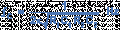However, i made my transfer function too complicated and am kinda stuck. Anyone have any idea on how i should proceed or did i do some steps wrongly? Thank you for your time.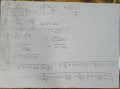#### The Electrician

Joined Oct 9, 2007
2,924

#### neonstrobe

Joined May 15, 2009
185
You are working along the right lines, but your Zth is upside down. You forgot the ^-1 I think.
Rather than substituting the impedances "as is" and ending up with a complicated expression, try re-doing but expanding the inverse terms as you go. If you keep multiplying out by the denominators you should be able to keep things a little tidier.
I haven't checked further but you may have things wrong because of the first problem.
But as an example, you could multiply by R3 to eliminate one denominator in your last line.

#### Wompalamba

Joined Dec 28, 2020
19
@The Electrician
Thank you for that paper. I now understand that i need to square the s and then square root to get the angular frequency polynomial term. And then set the polynomial equal to zero to solve for the cut off frequency. However, i am currently having issue trying to simplify the equation into a more workable form.

@neonstrobe
I have corrected the mistake you pointed out and tried to reduce the equation by eliminating the denominator. However i am currently stuck after eliminating the the first denominator. Is it not possible to simplify this further ?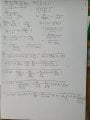#### The Electrician

Joined Oct 9, 2007
2,924
Of course it's possible to simplify more. You just have to keep slogging away at it. It's easy to make a mistake doing all that algebra. Have you ever used a modern mathematical software such as Matlab, Mathcad, Mathematica, Maple, etc., or the online version of Mathematica: https://www.wolframalpha.com/

I will tell you that you have your first expression for Vout/Vin correct, but I haven't checked all the algebra that follows.

Getting all your denominators eliminated is just the first step. Then you have to calculate the complex conjugate and find roots of the 4th degree polynomial (with constant term made negative) that results and pick the largest.

Are you required to end up with a symbolic expression for Fo? It would be a lot easier to substitute numerical values for the circuit components into your first expression for Fo and then crunch the numbers.

I used Mathematica to get a final symbolic result for Fo and it's a mess!#### Wompalamba

Joined Dec 28, 2020
19
Ah i was trying to decide on the component value for this filter design base on the final symbolic result. So it is more easier to tune the component value to a reasonable value so i can find an available component at the store. I obtain a copy of mathcad recently for this project but as i just started using it, i am still unfamiliar with the functions . To add on, i have tried to substitute in numbers for my component and i suspect i understood the website you provide wrongly as i got a negative number for my resistor. Please let me know where i went wrong. Thank you!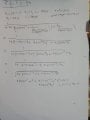#### LvW

Joined Jun 13, 2013
1,645
@The Electrician
And then set the polynomial equal to zero to solve for the cut off frequency.
Please, can you explain why? Is this in accordance with definition of the cut-off frequency?

#### ericgibbs

Joined Jan 29, 2010
17,426
hi W,

As a calculation reference check ONLY, this is the LTS result

Assumed R1=R2 =54.7Ω
E

#### Wompalamba

Joined Dec 28, 2020
19
Please, can you explain why? Is this in accordance with definition of the cut-off frequency?
According to the paper, http://www.crbond.com/papers/ent2-4.pdf . it is to find the maximum real zero for the pole.
I am still unsure of which step in my calculation i went wrong.

#### The Electrician

Joined Oct 9, 2007
2,924
Ah i was trying to decide on the component value for this filter design base on the final symbolic result. So it is more easier to tune the component value to a reasonable value so i can find an available component at the store. I obtain a copy of mathcad recently for this project but as i just started using it, i am still unfamiliar with the functions . To add on, i have tried to substitute in numbers for my component and i suspect i understood the website you provide wrongly as i got a negative number for my resistor. Please let me know where i went wrong. Thank you!View attachment 227234
Is this actual homework or are you self studying?

Is your desired value for Fo (you denoted it just F) 100 kHz or 900 kHz?

You can't get the 4th degree polynomial by just doubling the exponents of your transfer function denominator. You must replace "s" with "jw" and calculate the product of the denominator and its complex conjugate. This will give the desired 4th degree polynomial, then changing the sign of the constant part and calculating the largest real root will give you Fo.

Last edited:

#### Wompalamba

Joined Dec 28, 2020
19
Is this actual homework or are you self studying?

Is your desired value for Fo (you denoted it just F) 100 kHz or 900 kHz?

You can't get the 4th degree polynomial by just doubling the exponents of your transfer function denominator. You must replace "s" with "jw" and calculate the product of the denominator and its complex conjugate. This will give the desired 4th degree polynomial, then changing the sign of the constant part and calculating the largest real root will give you Fo.
I wish this were homework at least i have the answers to work backwards from. This is a project i am working on but i am not very proficient in filter design . 900kHz is my cut off frequency.

Is this step correct to find the complex conjugate?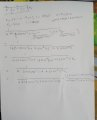#### ericgibbs

Joined Jan 29, 2010
17,426
Hi W,
If this project you are working on is not a Homework study assignment, why are you doing it the hard by using a transfer function method.?

If you just need a 3dB cut off at 900kHz there are number of online calculators, also LTSpice could give you the answer.

E

#### Wompalamba

Joined Dec 28, 2020
19
Hi W,
If this project you are working on is not a Homework study assignment, why are you doing it the hard by using a transfer function method.?

If you just need a 3dB cut off at 900kHz there are number of online calculators, also LTSpice could give you the answer.

E
I am not sure how to select the correct component to get the cut off without using guess and check if i am using LTSpice to get the cut off frequency. Most online calculator i found on this have preset circuit that are not similar to mine. But I might have miss out on some , do you have any recommendation on a better way to tackle this? Thank you.

#### ericgibbs

Joined Jan 29, 2010
17,426
hi W,
The circuit you posted just needs a change in the cap values.
You have said in the other thread you want 50R Impedances In and Out.

Look at this simulation.
Do you use LTS.?
E

BTW: Could you explain where the circuit is being used and how in the application.?

#### The Electrician

Joined Oct 9, 2007
2,924
I wish this were homework at least i have the answers to work backwards from. This is a project i am working on but i am not very proficient in filter design . 900kHz is my cut off frequency.

Is this step correct to find the complex conjugate?View attachment 227253
To calculate the complex conjugate you must replace "s" by "jw".

Here's how I calculated the transfer function and used a built-in command in Mathematica to put everything over a common denominator.

The first big fraction in the brackets calculates Vx/Vin, the second smaller fraction calculates Vout/Vx, and of course, the product of the two is Vout/Vin. The "Together" command puts it all over a common denominator.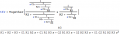Without showing all the intermediate steps in deriving the product of the denominator with its complex conjugate, and finding the largest real root with the constant part of the 4th degree polynomial negated, here is the expression for Fo. I've set the component values to numerical quantities and evaluated the expression for Fo giving a result of 900.515 kHz:•Wompalamba

#### Wompalamba

Joined Dec 28, 2020
19
hi W,
The circuit you posted just needs a change in the cap values.
You have said in the other thread you want 50R Impedances In and Out.

Look at this simulation.
Do you use LTS.?
E

BTW: Could you explain where the circuit is being used and how in the application.?
Yes i do use LTSpice to confirm my calculation after finding the transfer function. I am actually trying to make a second order RC circuit but i combine the input impedance with the first resistor to make the calculation easier but that failed. The infomation i was given was that this circuit will be use to transmit a AC signal source which need to have little to no attenuation between 100kHz to 200kHz which is the working frequency/bandwidth as well as filtering of the signal beyond that frequency which is why i set my cut off frequency at 900kHz. This is also the reason why i did not consider circuit with inductor in series.#### Wompalamba

Joined Dec 28, 2020
19
To calculate the complex conjugate you must replace "s" by "jw".

Here's how I calculated the transfer function and used a built-in command in Mathematica to put everything over a common denominator.

The first big fraction in the brackets calculates Vx/Vin, the second smaller fraction calculates Vout/Vx, and of course, the product of the two is Vout/Vin. The "Together" command puts it all over a common denominator.

View attachment 227261

Without showing all the intermediate steps in deriving the product of the denominator with its complex conjugate, and finding the largest real root with the constant part of the 4th degree polynomial negated, here is the expression for Fo. I've set the component values to numerical quantities and evaluated the expression for Fo giving a result of 900.515 kHz:

View attachment 227262
Wow thank you! That is a very complicated function . Is Mathematica able to help derive the complex conjugate or all the intermediate steps must be done manually?

#### ericgibbs

Joined Jan 29, 2010
17,426

#### Attachments

• 1 KB Views: 2
• 56.4 KB Views: 7
Last edited:

#### Wompalamba

Joined Dec 28, 2020
19
hi W,
As you use LTS, this is a asc file.
E
Thank you. How did you know what to choose for the value for the component ?

#### ericgibbs

Joined Jan 29, 2010
17,426
hi W,
•